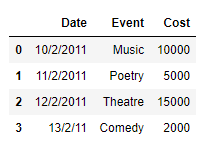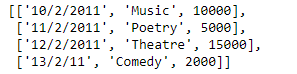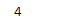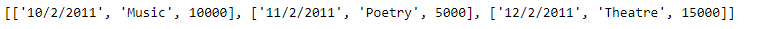# Create a list from rows in Pandas DataFrame | Set 2

• Last Updated : 29 Jan, 2019

In an earlier post, we had discussed some approaches to extract the rows of the dataframe as a Python’s list. In this post, we will see some more methods to achieve that goal.

Solution #1: In order to access the data of each row of the Pandas dataframe, we can use `DataFrame.iloc` attribute and then we can append the data of each row to the end of the list.

 `# importing pandas as pd``import` `pandas as pd`` ` `# Create the dataframe``df ``=` `pd.DataFrame({``'Date'``:[``'10/2/2011'``, ``'11/2/2011'``, ``'12/2/2011'``, ``'13/2/11'``],``                    ``'Event'``:[``'Music'``, ``'Poetry'``, ``'Theatre'``, ``'Comedy'``],``                    ``'Cost'``:[``10000``, ``5000``, ``15000``, ``2000``]})`` ` `# Print the dataframe``print``(df)`

Output :Now we will use the `DataFrame.iloc` attribute to access the values of each row in the dataframe and then we will construct a list out of it.

 `# Create an empty list``Row_list ``=``[]`` ` `# Iterate over each row``for` `i ``in` `range``((df.shape[``0``])):`` ` `    ``# Using iloc to access the values of ``    ``# the current row denoted by "i"``    ``Row_list.append(``list``(df.iloc[i, :]))`` ` `# Print the list``print``(Row_list)`

Output :As we can see in the output, we have successfully extracted each row of the given dataframe into a list. Just like any other Python’s list we can perform any list operation on the extracted list.

 `# Find the length of the newly ``# created list``print``(``len``(Row_list))`` ` `# Print the first 3 elements``print``(Row_list[:``3``])`

Output :Solution #2: In order to access the data of each row of the Pandas dataframe we can use `DataFrame.iat` attribute and then we can append the data of each row to the end of the list.

 `# importing pandas as pd``import` `pandas as pd`` ` `# Create the dataframe``df ``=` `pd.DataFrame({``'Date'``:[``'10/2/2011'``, ``'11/2/2011'``, ``'12/2/2011'``, ``'13/2/11'``],``                    ``'Event'``:[``'Music'``, ``'Poetry'``, ``'Theatre'``, ``'Comedy'``],``                    ``'Cost'``:[``10000``, ``5000``, ``15000``, ``2000``]})`` ` `# Create an empty list``Row_list ``=``[]`` ` `# Iterate over each row``for` `i ``in` `range``((df.shape[``0``])):``    ``# Create a list to store the data``    ``# of the current row``    ``cur_row ``=``[]``     ` `    ``# iterate over all the columns``    ``for` `j ``in` `range``(df.shape[``1``]):``         ` `        ``# append the data of each``        ``# column to the list``        ``cur_row.append(df.iat[i, j])``         ` `    ``# append the current row to the list``    ``Row_list.append(cur_row)`` ` `# Print the list``print``(Row_list)`

Output :`# Find the length of the newly ``# created list``print``(``len``(Row_list))`` ` `# Print the first 3 elements``print``(Row_list[:``3``])`

Output :My Personal Notes arrow_drop_up